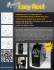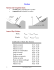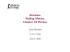# Exam 2 Solutions

## Transcription

Exam 2 Solutions
```Phsics 2210
Spring 2015
Dr. Charlie Jui
Name:
EXAM
Unid:
Discussion TA:
' CX
A spring offeree constant & = 800 N/m and a relaxed length L 0 = 1.10m has its upper
end attached to a pivot in the ceiling. Its free end is attached to a block of mass
M = 45.0 kg that sits on a frictionless, horizontal surface. When the block is directly
below the pivot, the spring is stretched to a length h = 1.20 m. The block is then pulled
left a horizontal distance b = 0.90 m and released from rest. Calculate
(a)
(b)
(c)
the magnitude of the force exerted by the spring on the block,
the acceleration of block right after being released, and
the speed of block as it passes directly under the pivot again.
You can assume that the block remains on the surface.
jr_- fc.M/J « K>(L-U) *
( Hood's
"* 32o A/
p
I XX
H
_
£.c«*
M
..c^^rc*«n/i) ) .i
^
V» ' /
tt
^
y,y
/
1
Spring 2015
Physics 2210
Dr. Charlie Jui
Name:
EXAM 2
Unid:
Discussion TA:
In the figure, the pulley has negligible mass and is frictionless. Block A has a mass
of 2.5 kg, and block B 4.0 kg. The angle of the incline is 9 = 35°. The coefficient of
kinetic friction between the incline and block A is /^ = 0.30. The blocks are set in
motion from rest with the cord taut. Find
(a)
the magnitude of the friction force exerted by the incline on block A.
After block B falls a distance of 45 cm, calculate
(b)
(c)
the work done by gjavity on block A, and
the total kinetic energy of the two blocks.
You can assume that block A remains on the incline.
~
=>
2
Dr. Charlie Jui
Spring 2015
Physics 2210
Z IvUsXf
Name:
3
EXAM 2
Unid:
Discussion TA:
A block in the figure has mass m-^ = 6.10 kg. The coefficient of static
friction between the block and the horizontal desk top is /j.s = 0.40. The
block is attached by a cord to a cowbell of mass m2 = 1.25kg. The
cowbell is attached by a second cord to a hook on the wall. The first cord
is horizontal, and the second cord at an angle of 8 = 40.0° from the
horizontal.
(a)
(b)
(c)
?T?
"1
The system is in equilibrium as shown. Calculate the tension T2
Calculate the magnitude of the friction force exerted by the desk on the block.
How much additional mass can be added to the cowbell (i.e. to m2) and still keep the system in equilibrium?
-0
rt
6jv = »
d
is/
T,
\
```

### 550 CORD/PARACHUTE CORD CUT TO 5" AND MELT THE ENDS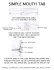### Ms. Mulrooney Physics Worksheet: Coefficients of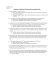### Free-Body Diagram Worksheet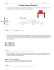### WARNING: The Manitou Incline is an EXTREME trail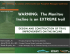### Definitions - New England Cord Blood Bank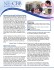### I`ll Fly Away: TUESDAY, SEPT. 15 • 7 P.M.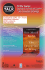### GO ON TO THE NEXT PAGE. Section I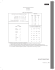### Social Justice and Human Rights Anthon Heyns Bioethics Forum 15 June 2007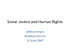### Easy Reel spooler